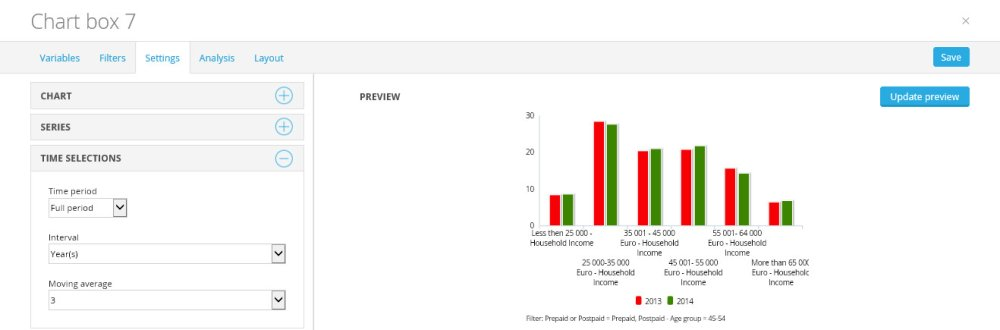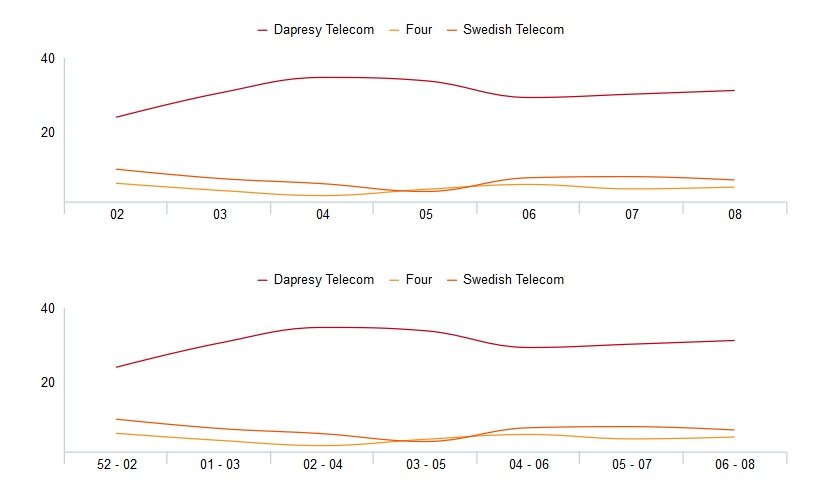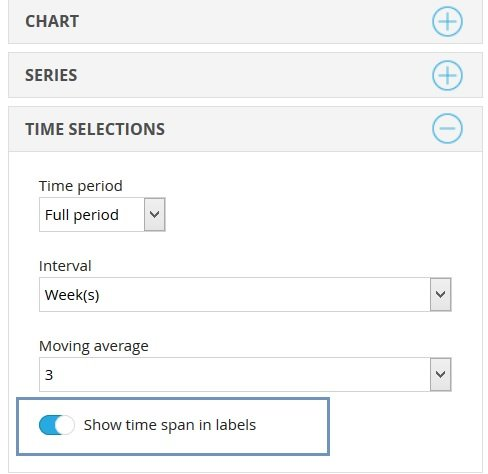The time selection options are placed after the series settings in the Time selection menu, as shown in the image below. The time selection will determine what period we are charting and with the change of Interval our Interval that we are showing in Legend (or Axis) will also change. Remember that with changing the Time selection you need to save for changes to take place.Another option here is Moving average. Here is the example of how this option works: If for example you have 10 points in a series. It will take sum of the first point + sum of the second point + sum of the third point and divide it with respondents in 1 and 2 and 3. This value will be the first showing point in the series. For the second point it will take 2+3+4 and calculate an average for that point.

Note: You can select to use up to 53 periods in the moving average calculation.A last control button within the Time selection lets you show the time span within the labels when using a Moving Average calculation. By turning on the settings for label logic, it’s much clearer to tell which dates each period is based upon, making the reports more user-friendly. The charts below show a 3-week moving average. The first chart uses the label logic without showing the times span, and the second chart uses the label logic on the same chart, but with the time span control turned on. After turning on the moving average, you'll have the option to show the first intervals (even though they are not calculated based on the complete number of intervals. By default those will be hidden.To use this option just select the control “Show time span in labels” shown in the image below.